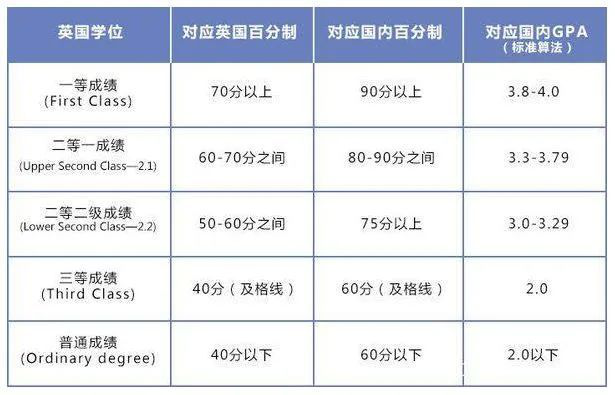GPA计算方法

(1)、加权平均

(2)、平均成绩(最简单算法)211大学的学生：

A=100-90=4.0
B=89-80=3.0
C=79-70=2.0
D=69-60=1.0
F=低于60=0.0

A课程四个学分，成绩92(A);
B课程三个学分，成绩80(B);
C课程两个学分，成绩98(A);
D课程六个学分，成绩70(C);
E课程三个学分，成绩89(B)。

GPA标准算法：
[(92×4+80×3+98×2+70×6+89×3)×4]/[(4+3+2+6+3)×100]=3.31

GPA常见算法：
(4×4+3×3+2×4+6×2+3×3)/(4+3+2+6+3)=3.00

4.33分制（西蒙菲莎大学）

12分制 （卡尔顿大学）

4分制（麦吉尔大学）